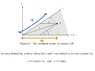# Coordinates transformation

unscientific

## Homework Statement

The x-y coordinates are being transformed into the u-v coordinates.

Based on the diagram, u lies along x while v makes an angle α with x.

## The Attempt at a Solution

The answer defined u and v weirdly..

Shouldn't

x = u

and

y = v sin α

??

#### Attachments

•coordinate.jpg
14.5 KB · Views: 359

Homework Helper
Gold Member
Shouldn't

x = u

and

y = v sin α

??

No. Consider the position vector $\mathbf{r}= x\mathbf{e}_x + y\mathbf{e}_y = u\mathbf{e}_u + v\mathbf{e}_v$:

You have $\mathbf{e}_u = \mathbf{e}_x$ since the two axes are parallel, but $\mathbf{e}_v$ has both a vertical and a horizontal component and is given by $\mathbf{e}_v = \cos\alpha \mathbf{e}_x + \sin\alpha \mathbf{e}_y$. Plugging this into the position vector definition gives $x\mathbf{e}_x + y\mathbf{e}_y= u \mathbf{e}_x + v( \cos\alpha \mathbf{e}_x + \sin\alpha \mathbf{e}_y)$, which gives you the relations in your image.

Homework Helper
Gold Member
According to the answer, the region R → R', where R' is only σ/2∏ of the original R..

No, that's not what they are saying. Read it again more carefully, what they are actually claiming is that $\int_{0}^{\infty} \int_{0}^{\infty} e^{-r^2} \left| \frac{\partial(x,y)}{\partial(u,v)} \right|dudv = \frac{\alpha}{2\pi} \int_{-\infty}^{\infty} \int_{-\infty}^{\infty} e^{-r^2}dxdy$

The integral on the left is only over positive $u$ & $y$, while the integral on the right is over all (R2) space.

unscientific
No, that's not what they are saying. Read it again more carefully, what they are actually claiming is that $\int_{0}^{\infty} \int_{0}^{\infty} e^{-r^2} \left| \frac{\partial(x,y)}{\partial(u,v)} \right|dudv = \frac{\alpha}{2\pi} \int_{-\infty}^{\infty} \int_{-\infty}^{\infty} e^{-r^2}dxdy$

The integral on the left is only over positive $u$ & $y$, while the integral on the right is over all (R2) space.

Yup, if you only consider x,y,u,v > 0,

it would be α/(∏/2) for ∫ 0 to infinity..

unscientific
No. Consider the position vector $\mathbf{r}= x\mathbf{e}_x + y\mathbf{e}_y = u\mathbf{e}_u + v\mathbf{e}_v$:

You have $\mathbf{e}_u = \mathbf{e}_x$ since the two axes are parallel, but $\mathbf{e}_v$ has both a vertical and a horizontal component and is given by $\mathbf{e}_v = \cos\alpha \mathbf{e}_x + \sin\alpha \mathbf{e}_y$. Plugging this into the position vector definition gives $x\mathbf{e}_x + y\mathbf{e}_y= u \mathbf{e}_x + v( \cos\alpha \mathbf{e}_x + \sin\alpha \mathbf{e}_y)$, which gives you the relations in your image.

I don't really understand what you mean...

My main problem here is why do they define u and v as such in the picture?

I thought u and v are defined when you drop a perpendicular line onto the axis?

And it's pretty clear that the lengths u, v they define are shorter than the ones in my picture..

voko
I thought u and v are defined when you drop a perpendicular line onto the axis?

That's the case when your coordinate lines are perpendicular. When they are not, you get what you see in this picture.

unscientific
That's the case when your coordinate lines are perpendicular. When they are not, you get what you see in this picture.

Are they defined this way?

voko
A coordinate system (on an plane) is defined by its origin and unit vectors $\vec{a}$ and $\vec{b}$. Any point $\vec{p} = u\vec{a} + v\vec{b}$. $u$ and $v$ are coordinates. Now if the coordinate unit vectors are not perpendicular, what do you get? Try it on a piece of paper.

unscientific
A coordinate system (on an plane) is defined by its origin and unit vectors $\vec{a}$ and $\vec{b}$. Any point $\vec{p} = u\vec{a} + v\vec{b}$. $u$ and $v$ are coordinates. Now if the coordinate unit vectors are not perpendicular, what do you get? Try it on a piece of paper.

Ah, using vectors everything seems much simpler now! Thank you!